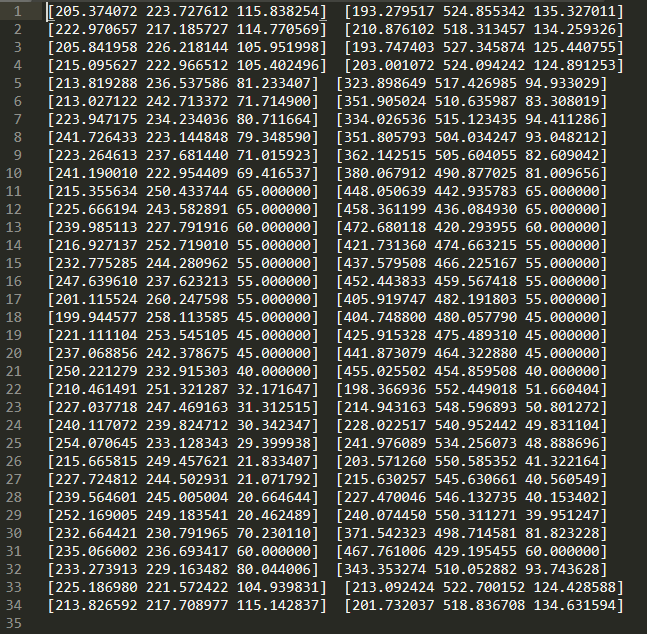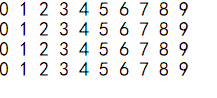• C++读取文本文件 比如有一批坐标点： 我想读取进一个vector数组中，代码片如下： const char* FileName = "./Resources/Plan.txt"; ifstream readFile; readFile.open(FileNeedlePos, ios::in); vector<float>...
C++读取文本文件
比如有一批坐标点：我想读取进一个vector数组中，代码片如下：
const char* FileName = "./Resources/Plan.txt";
ifstream readFile;
readFile.open(FileNeedlePos, ios::in);
vector<float> pos;
string str;
while (readFile >> str)
{
str.erase(remove(str.begin(), str.end(), '['), str.end());
str.erase(remove(str.begin(), str.end(), ']'), str.end());
pos.push_back(stof(str));
cout << str << endl;
}
readFile.close();



展开全文• 头文件 #include <fstream> 1.按字符读取 void readtxt() { ... // 打开文本文件 指针指向文件开头 char ch; while (EOF != (ch = f.get())) //EOF 是-1 即读入的已不是正常的字符而是...
头文件
#include <fstream>

1.按字符读取
void readtxt()
{
ifstream f;   //实例一个输入流指针
f.open("text.txt", ios::in);  // 打开文本文件  指针指向文件开头
char ch;
while (EOF != (ch = f.get()))  //EOF 是-1 即读入的已不是正常的字符而是文件的结束符
cout << ch;
}


2.按行读取
void readtxtbyline ()
{
ifstream f;   //实例一个输入流指针
f.open("text.txt", ios::in);  // 打开文本文件  指针指向文件开头
char line;
f.getline(line, 6);		//getline（line,6）  line是前面定义好的数组首地址，6是文本文件中这一行要读取的字符数，要保留前3个数 就输入3
cout << line << endl;
cout << line << endl;  //line  就是和前面定义的数组有关，当指针指向数组首地址时，数组的内容就按照文本文件内容顺延
f.getline(line, 6);		//输入流指针会自动移到下一行
cout << line << endl;
f.seekg(0, 0);		//  设定指针位置  (0,0)指针设定在文件开头     (0,ios::end)指针设定在文件结尾
while (!f.eof())	//使用eof()函数检测文件是否读结束
{
f.getline(line, 128);
cout << line << endl;
}
}




展开全文• #include <fstream> std::string loadFile(const std::string& file) { std::string content; if (!file.empty()) { std::ifstream stream(file); if (!stream.is_open()) { std::cerr <...&.
#include <fstream>
std::string loadFile(const std::string& file)
{
std::string content;
if (!file.empty()) {
std::ifstream stream(file);
if (!stream.is_open()) {
std::cerr << "Failed to open file " << file << std::endl;
return false;
} else {
content = std::string((std::istreambuf_iterator<char>(stream)),
(std::istreambuf_iterator<char>()));
stream.close();
}
}
return content;
}


展开全文• ## C++读取文本文件的几种方法

千次阅读 多人点赞 2019-12-19 17:39:08
前几天要用到C++读取文本文件，就学习了一下几种不同的读取方法: 文本文件内容如下： 第一种：直接读取，以空格换行 int main（） { ifstream infile; infile.open("qqzl.txt", ios::in); if (!infile.is_open...
前几天要用到C++读取文本文件，就学习了一下几种不同的读取方法:
文本文件内容如下：第一种：直接读取，以空格换行
	int main（）
{
ifstream infile;
infile.open("qqzl.txt", ios::in);
if (!infile.is_open())
{
cout << "读取文件失败" << endl;
return;
}
//第一种读取方法，
char buf = { 0 };
while (infile>>buf)
{
cout << buf << endl;//输出读取的文本文件数据
}
}


第二种：数组方法，逐行读取，可读取空格
	int main（）
{
ifstream infile;
infile.open("qqzl.txt", ios::in);
if (!infile.is_open())
{
cout << "读取文件失败" << endl;
return;
}
//第二种读取方法
char buf;
while (infile.getline(buf,sizeof(buf)))
{
cout << buf << endl;
}
}

第三种：字符串读取，逐行读取，可读取空格
	int main（）
{
ifstream infile;
infile.open("qqzl.txt", ios::in);
if (!infile.is_open())
{
cout << "读取文件失败" << endl;
return;
}
//第三种读取方法
string buf;
while (getline(infile,buf))
{
cout << buf << endl;
}
}

第四种：逐字符读取，可读取空格，但是效率较低
	int main（）
{
ifstream infile;
infile.open("qqzl.txt", ios::in);
if (!infile.is_open())
{
cout << "读取文件失败" << endl;
return;
}
//第四种读取方法
char c;
while ((c=infile.get())!=EOF)
{
cout << c;
}
}

第五种：读取至Vector容器中
	int main（）
{
ifstream infile;
infile.open("qqzl.txt", ios::in);
if (!infile.is_open())
{
cout << "读取文件失败" << endl;
return;
}
//第五种读取方法
string s;
vector<string>v1;
while (getline(infile,s))
{
infile >> s;
v1.push_back(s);
}
for (int i = 0; i < v1.size(); i++)
{
cout << v1.at(i);
cout << endl;
}
infile.close();
}

这是暂时总结的几种方法，就当记录一下。


展开全文• C++读取文本文件内容到char*中： #include <iostream> #include <fstream> #include <iostream> const char* readFile(const char* fileName); int main() { const char* result = readFile...字符串 char strcpy
• 在OSG环境下用C++编写的可以读取文本文件数据的代码
• 最近在做一个东东，遇到文件读取的问题，遂开始研究，使用C++读取文本文件比较简单，也是一个很好的切入点，所以进行了一些小实验。 这里仅仅举例，使用ANSI,UNICODE,UTF-8三种常用的文本文件作为示例。 ...
•   这篇博客是一个c++读取txt文件的代码，当然，其他后缀的文本文件啥的读取方法也是一样的，如果是二进制文件那就需要改一下读取模式了。写这篇博客主要是因为有时候会需要简单一个小程序处理一下数据什么的，但是...
• C++代码，如何一次读取文件所有内容？ 代码示例如下： #include <fstream> #include <string> int main(int argc, char* argv[]) { std::ifstream inputStream(argv); const std::string text(...
• #include <iostream> #include <fstream> #include <cstdlib> using namespace std; const int SIZE = 60; int main() { char filename[SIZE]; ifstream inFile;... inFile.ope
• 有时候我们需要自定义文件内容格式，然后从文本文件读取数据或控制信息，在程序里面进行相应的处理。例如用文本文件存储空间点的坐标，设定文件的每一行有三个数，分别表示x y z，比如 0 0 0 1 2 3 2 3 3 表示(0......

# c++读取文本文件c++ 订阅